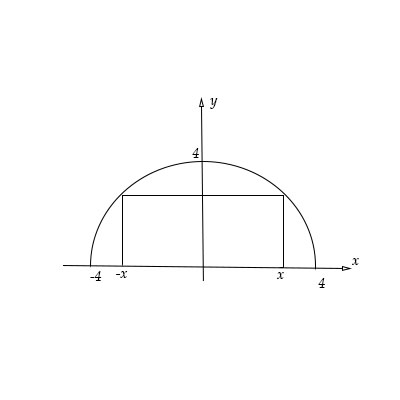# A rectangle is to be inscribed in a semicircle y= \sqrt {16-x^2} of radius 4. Find the dimension...

## Question:

A rectangle is to be inscribed in a semicircle {eq}y= \sqrt {16-x^2} {/eq} of radius {eq}4 {/eq}. Find the dimension of the rectangle with largest area.## Maxima/Minima of a Function:

Given a function f(x), if it attains a maximum or minimum at a point a, then {eq}\frac{df}{dx} |_{x=a} =0 {/eq}

To know whether the point gives a maximum or a minimum, we can apply the second derivative test at that point. If the second derivative at that point is negative, then the point corresponds to a maximum. If the second derivative at the point is positive, then it corresponds to a minimum.

Take any arbitrary x position. The dimensions of the inscribed rectangle will be {eq}(2x, y) {/eq}

Area of the rectangle will be :

{eq}A = 2xy = 2x\sqrt{16-x^2} {/eq}

To maximise the area :

{eq}\frac{dA}{dx} = 0 \\ 2\sqrt{16-x^2} + 2x(\frac{-2x}{2\sqrt{16-x^2}}) = 0 \\ 2(16-x^2) - 2x^2 = 0 \\ 32 - 4x^2 = 0 \\ x= \pm 2\sqrt 2 \\ y=16 {/eq}

The dimensions of the rectangle with largest area are :

{eq}4\sqrt 2 \times 16 {/eq}